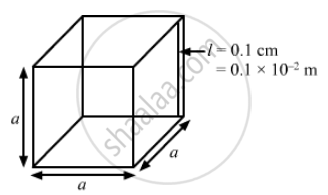Advertisement Remove all ads

# A Cubical Box of Volume 216 Cm3 is Made up of 0.1 Cm Thick Wood. the Inside is Heated Electrically by a 100 W Heater. It is Found that the Temperature Difference Between the Inside and the Outside - Physics

Sum

A cubical box of volume 216 cm3 is made up of 0.1 cm thick wood. The inside is heated electrically by a 100 W heater. It is found that the temperature difference between the inside and the outside surface is 5°C in steady state. Assuming that the entire electrical energy spent appears as heat, find the thermal conductivity of the material of the box.

Advertisement Remove all ads

#### SolutionVolume of the cube, V = a3 = 216 cm3
Edge of the cube, a = 6 cm
Surface area of the cube = 6a
= 6 (6 × 10−2)2
= 216 × 10−4 m2
Thickness, l = 0.1 cm = 0.1 × 10–2 m
Temperature difference, Δ T = 5°C
The inner surface of the cube is heated by a 100 W heater.
∴ Power, P = 100 W
Power = Energy per unit time
∴ Rate of flow of heat inside the cube, R = 100 J/s
Rate of flow of heat is given by
(DeltaQ)/(Deltat) = (DeltaT)/(l/(KA)

100 = Kxx216xx10^-4xx5/(0.1xx10^-2)

K = 0.9259  W/ m° C

Is there an error in this question or solution?
Advertisement Remove all ads

#### APPEARS IN

HC Verma Class 11, Class 12 Concepts of Physics Vol. 2
Chapter 6 Heat Transfer
Q 12 | Page 99
Advertisement Remove all ads
Advertisement Remove all ads
Share
Notifications

View all notifications

Forgot password?